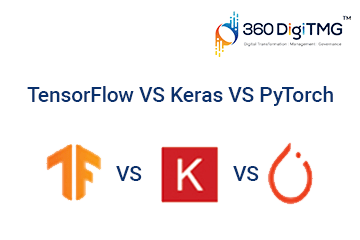Home / Blog / Artificial Intelligence / Loss (Error) Functions in Machine Learning

# Loss (Error) Functions in Machine Learning

• July 12, 2023
• 12203
• 40### Meet the Author : Mr. Bharani Kumar

Bharani Kumar Depuru is a well known IT personality from Hyderabad. He is the Founder and Director of Innodatatics Pvt Ltd and 360DigiTMG. Bharani Kumar is an IIT and ISB alumni with more than 17 years of experience, he held prominent positions in the IT elites like HSBC, ITC Infotech, Infosys, and Deloitte. He is a prevalent IT consultant specializing in Industrial Revolution 4.0 implementation, Data Analytics practice setup, Artificial Intelligence, Big Data Analytics, Industrial IoT, Business Intelligence and Business Management. Bharani Kumar is also the chief trainer at 360DigiTMG with more than Ten years of experience and has been making the IT transition journey easy for his students. 360DigiTMG is at the forefront of delivering quality education, thereby bridging the gap between academia and industry.

The optimum model for machine learning is the one that obtains the greatest or lowest score, accordingly. Machine learning may be viewed as an optimisation issue where an objective function needs to be either maximised or minimised.

In machine learning challenges, the goal is often to reduce the difference between the projected value and the actual value. Losses or errors refer to the cost associated with failing to provide the desired results. It is referred to as a loss or error function if the loss is determined for just one training sample. The loss is referred to as a cost function if it is averaged over the full training sample.

Loss functions change depending on the kind of issue we are attempting to resolve. Classification problems, in which the algorithm tries to classify the training sample into one of the target classes, have a different set of loss/cost functions than regression problems, which aim to predict a continuous value. Let's examine some of the cost functions that are most frequently employed in machine learning algorithms.

Are you looking to become a Machine Learning Engineer? Go through 360DigiTMG's Machine Learning Certification in Bangalore.

## Mean Error Loss:

As the name suggests it is the average of all errors in a set. The ‘error’ is defined as the difference between the predicted value and actual value. It is also called ‘observational’ or ‘measurement’ error.

ME = sum of all values in the data / number of values in data.

This is not a preferred method because there is a chance that the positive and negative values cancel each other giving an illusion of no error.

## Mean Squared Error/ Quadratic Loss/ L2 Loss:

It is mathematically represented as: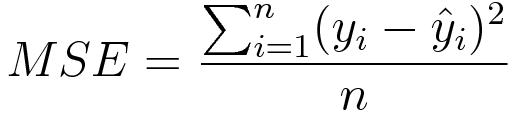The average squared difference between the anticipated values and actual values serves as the loss function in this, one of the most used cost functions. The direction of the mistake is irrelevant since the difference is squared; only the amount counts. Additionally, it is simpler to determine the gradient of the cost function.

## Mean Absolute Error/ L1 Loss:

The mathematical representation is :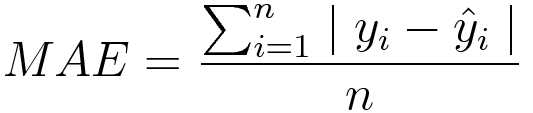The mean of the absolute disparities between the predicted and actual values makes up the mean absolute error. It and MSE are comparable in that only the magnitude, not the direction, is important to this cost function. The calculation of gradients is little more difficult than with MSE since we must use methods from linear programming to do so.

## Mean Squared Logarithmic Error Loss (MSLE):

The mathematical representation of MSLE is as shown below:

According to the understanding, MSLE measures the disparity between observed values that are actual and those that are expected. By just considering the percentage difference between the actual and projected values, MSLE avoids overly harshly penalising significant errors (which is what the MSE function frequently does). This is especially helpful when the target variable has a large range of values, some of which may be many orders of magnitude greater than the mean due to the commercial use case. Although the figures are totally respectable, they are often seen as outliers. Housing costs are a common example, where individual homes may be several orders of magnitude more expensive than the average cost of a home in that location.

## Mean Percentage Error:

Mean percentage error is basically the average of the percent errors of the differences between predicted and actual values.The mathematical representation is:

The problem with this error is that it is undefined when a value becomes zero.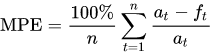## Mean Absolute Percentage Error:

This function is known by another name - Mean Absolute Percentage Deviation,which is a calculation of the average of absolute percent of errors. The mathematical formulation is:

yourself a promising career in Machine Learning Training center in Chennai by enrolling in the Machine Learning Program offered by 360DigiTMG.

MAPE is one of the most commonly used loss functions in regression analysis and also in evaluating the model as it is highly intuitive because it is very easily interpreted in terms of the relative error.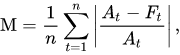## Classification Loss Functions:

#### Binary Classification LossesBinary Cross Entropy:

Binary cross entropy is the measure of the difference between the probability distributions for a set of given random variables and/or events.In the case of a two class Classification, target variables are have two classes and the cross-entropy can be defined as: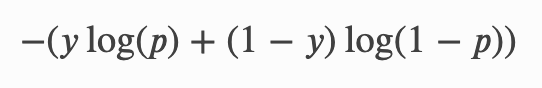## Hinge Loss:

This loss typically serves as an alternative to the cross-entropy and was initially developed to use with the support vector machine algorithm. It typically works best when the values of the output variable are in the set of {-1, 1}. The mathematical representation of hinge loss is shown below: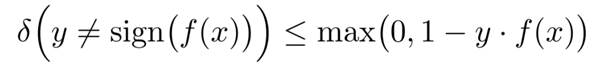## Squared Hinge Loss:

This is only the square of the hinge loss function and is an extension of the hinge loss. The fact that this is a square of the initial loss gives it some mathematical characteristics that make calculating the gradients simpler. This is ideal for queries of the Yes-or-No variety when the probability deviation is unimportant.

## Gini Impurity:

This loss function is used by the Classification and Regression Tree (CART) algorithm for decision trees. This is a measure of the likelihood that an instance of a random variable is incorrectly classified per the classes in the data provided the classification is random. The lower bound for this function is 0. For a set of items with J classes, the Gini impurity is shown below: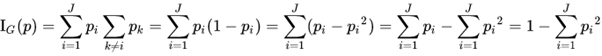## Hellinger Distance:

This is a cost function that satisfies the triangle inequality. For probability distributions P = {pi}i∈[n] , Q = {qi}i∈[n] supported on [n], the Hellinger distance between them is defined as:

The √ 2 in the definition is for ensuring that h(P, Q) ≤ 1 for all probability distributions.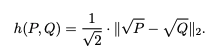## Itakura–Saito Distance:

It is a measure of the difference between original spectrum and an approximation of that spectrum as defined by the equation below: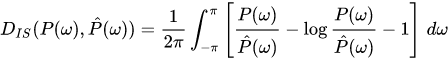## Multi-Class Classification Losses:

Also, check this Machine Learning Course Training in Pune to start a career in Machine Learning.

#### Multi-Class Cross-Entropy:

This is an extension of the binary cross-entropy calculation where the losses for each class are calculated separately added as the result. The mathematical representation of the multi-class cross-entropy is shown below:## Kullback Liebler (KL) Divergence:

The KL Divergence calculates the discrepancy between the anticipated and actual probability distributions. The distributions are said to be equal if the KL divergence score is 0.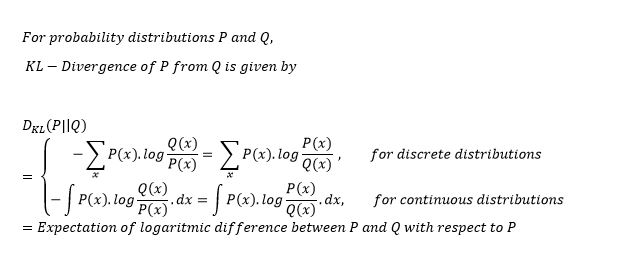### Macine Learning Training Institutes in Other Locations